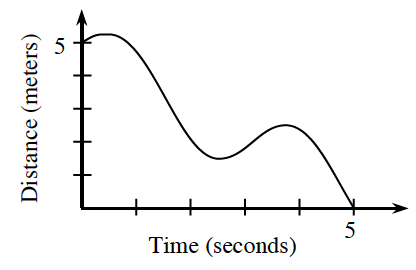### Home > APCALC > Chapter 2 > Lesson 2.3.2 > Problem2-115

2-115.

While running in a straight line to class, Steven’s distance from class (in meters) was recorded on the graph at right.

1. Estimate his velocity (in meters per second) at $t = 0, 1,$ and $3$ seconds.

Velocity is the steepness (or slope) of a distance graph. Since this graph is not linear, slope can only be approximated.

Think about ramp lab (2-112). Slope at a specific point was approximated by finding the slope of a line that connects two points close to the target.

We can approximate the slope at $t = 1$ by choosing two points close to $t = 1$ and finding the slope of the line that connects them.

$\text{velocity at } t = 1 \text{ is about } -\frac{5}{2} \text{ m/sec}$

2. Did Steven ever stop and turn around? If so, when? How does the graph show this?

This is a distance graph. $y$-values represent distance from the classroom.

3. Approximate the interval(s) of time when Steven was headed toward class.

The classroom is located at $y = 0$.